# Denoise EEG Signals Using Deep Learning Regression with GPU Acceleration

This example shows how to remove electro-oculogram (EOG) noise from electroencephalogram (EEG) signals using the EEGdenoiseNet benchmark dataset  and deep learning regression. The EEGdenoiseNet dataset contains 4514 clean EEG segments and 3400 ocular artifact segments that can be used to synthesize noisy EEG segments with the ground-truth clean EEG (the dataset also contains muscular artifact segments, but these will not be used in this example).

This example uses clean and EOG-contaminated EEG signals to train a long short-term memory (LSTM) model to remove the EOG artifacts. The regression model was trained with raw input signals and with signals transformed by the short-time Fourier transform (STFT). The STFT model improves performance especially at degraded SNR values.

To enable GPU acceleration for STFT computations, you must have Parallel Computing Toolbox™. To see which GPUs are supported, see GPU Computing Requirements (Parallel Computing Toolbox).

### Create the Dataset

The EEGdenoiseNet dataset contains 4514 clean EEG segments and 3400 EOG segments that can be used to generate three datasets for training, validating, and testing a deep learning model. The sample rate of all the signal segments is 256 Hz. For convenience, the dataset has been uploaded to this location: https://ssd.mathworks.com/supportfiles/SPT/data/EEGEOGDenoisingData.zip

Download the dataset using the `downloadSupportFile` function.

```% Download the data datasetZipFile = matlab.internal.examples.downloadSupportFile('SPT','data/EEGEOGDenoisingData.zip'); datasetFolder = fullfile(fileparts(datasetZipFile),'EEG_EOG_Denoising_Dataset'); if ~exist(datasetFolder,'dir') unzip(datasetZipFile,fileparts(datasetZipFile)); end```

After downloading the data, the location in `datasetFolder` contains two MAT files:

• `EEG_all_epochs.mat` contains a matrix with 4514 clean EEG segments of length 512 samples

• `EOG_all_epochs.mat` contains a matrix with 3400 EOG segments of length 512 samples

Use the `createDataset` helper function to generate training, validation, and testing datasets. The function combines clean EEG and EOG signals to generate pairs of clean and noisy EEG segments with different signal-to-noise ratios (SNR). For any EEG and EOG pair you can use the following combination equation to obtain a noisy segment with a given SNR:

`$\mathrm{noisyEEG}=\mathrm{EEG}+\lambda \cdot \mathrm{EOG}$`

`$\mathrm{SNR}=10\cdot {\mathrm{log}}_{10}\left(\frac{\mathrm{rms}\left(\mathrm{EEG}\right)}{\mathrm{rms}\left(\lambda \cdot \mathrm{EOG}\right)}\right)$`

You vary parameter $\lambda$ to control the artifact power and achieve a particular SNR value.

To create the training dataset `createDataset `combines the first 2720 pairs of EEG and EOG segments ten times each with random SNRs in the [-7, 2] dB interval for a total of 27200 training pairs. Each training pair is stored in a MAT file inside a folder named `train`. Each MAT file includes:

• A clean EEG segment (stored under a variable named `EEG`)

• An EOG segment (stored under a variable named `EOG`)

• A noisy EEG segment (stored under a variable named `noisyEEG`)

• The SNR of the noisy segment (stored under a variable named `SNR`)

• The sample rate value of the signal segments (stored under a variable named `Fs`)

To create the validation dataset `createDataset` combines the next 340 pairs of the EEG and EOG segments ten times each with random SNRs in the [–7, 2] dB interval for a total of 3400 validation segments. Validation data is stored in MAT files inside a folder named `validate`. Each MAT file contains the same variables as the ones described for the training set.

Finally, to create the test dataset `createDataset` combines the next 340 pairs of EEG and EOG segments ten times each with deterministic SNR values of –7, –6, –5, –4, –3, –2, –1, 0, 1, and 2 dB. The test data is stored in MAT files inside a folder named `test`. Test MAT files with the same SNR value are grouped under a common subfolder to make it easier to analyze the denoising performance of the trained model for a given SNR. For example, files with test signals with an SNR of -3 dB are stored in a folder with name `data_SNR_-3`.

Call the `createDataset` function to create the dataset (this may take a few seconds). Set the `createDatasetFlag` to false if you already have the dataset in the `datasetFolder` and want to skip this step.

```createDatasetFlag =true; if createDatasetFlag createDataset(datasetFolder); end```

### Prepare Datastores to Consume the Data

The generated dataset is quite large (~430 MB), so it is convenient to use datastores to access the data without having to read it all at once into memory. Create signal datastores to access the training and validation data. Use the `SignalVariableNames` parameter to specify the variables you want to read from the MAT files (in the order you want them read). Also specify the `ReadOutputOrientation` as "row" to ensure the data is compatible with the LSTM network.

```ds_Train = signalDatastore(fullfile(datasetFolder,"train"), ... SignalVariableNames=["noisyEEG","EEG"], ... ReadOutputOrientation="row"); ds_Validate = signalDatastore(fullfile(datasetFolder,"validate"), ... SignalVariableNames=["noisyEEG","EEG"], ... ReadOutputOrientation="row");```

Read the data from the first training file and plot the clean and noisy EEG signals. A call to preview or read methods of the datastore yields a 1x2 cell array with the first element containing a noisy EEG segment, and the second element containing a clean EEG segment.

`data = preview(ds_Train)`
```data=1×2 cell array {[211.6124 214.7588 70.1825 -28.2211 -147.5027 -227.7570 -278.1249 -323.9914 -394.9389 -447.8104 -485.9636 -479.6641 -341.6295 -131.7559 -10.5813 60.9458 215.0746 396.0737 509.4731 590.1085 635.1260 593.8397 539.2092 542.1175 494.7517 298.8678 48.4135 -134.4423 -258.3096 -357.4009 -403.8768 -406.9569 -438.5987 -502.7630 -537.9188 -545.7589 -554.1699 -540.9347 -526.4374 -570.8086 -626.8561 -598.1116 -483.5767 -323.4854 -162.3836 -71.2556 -24.3024 80.3198 182.8315 153.2236 61.2011 19.6223 -19.0150 -79.7520 -103.0416 -105.8191 -105.7669 -49.8751 11.4967 -30.1727 -126.5470 -178.1164 -195.2068 -202.2851 -204.3055 -236.4048 -284.3266 -306.8748 -358.1152 -463.6844 -492.6420 -359.1389 -160.2024 7.2763 155.2251 282.2257 329.2834 295.4677 265.4648 272.4693 253.7549 201.1175 181.7627 162.0763 59.4699 -34.7458 15.9173 127.8403 151.9076 129.0929 163.1323 236.8791 292.8300 313.1917 272.0181 174.0719 140.2538 267.9298 415.9153 381.7237 265.9353 301.8536 415.4972 405.1484 347.8450 374.5153 392.5318 360.6148 384.2820 432.2193 412.1695 391.7622 418.7219 424.0679 416.6217 454.8611 515.4259 569.4735 596.6404 557.9470 501.0518 488.2949 457.3884 393.8159 378.8010 348.0427 218.1068 142.3872 215.1134 249.9000 147.1429 47.7722 26.8799 22.2325 13.2872 44.4823 135.3305 207.4060 158.8223 73.6557 116.5746 203.6459 166.3977 96.5407 111.8745 107.1642 38.7024 28.4991 84.3733 113.4612 125.7470 155.6519 160.6718 126.2311 119.6526 180.1543 229.7300 201.5261 203.1078 304.4279 322.2615 197.7146 200.9325 352.7455 301.0753 55.2468 2.7528 154.5220 209.9317 157.3855 158.0628 182.0705 132.5070 42.1377 23.2491 99.8068 118.1615 -13.1857 -118.9997 -84.2277 -58.6776 -111.2676 -160.4009 -217.9776 -245.7238 -120.9668 29.8071 17.7024 -39.2196 -40.4029 -82.4125 -95.6460 20.1329 95.9889 69.8550 86.8802 65.4962 -103.2244 -217.8681 -203.1367 -242.5387 -308.0859 -299.5670 -350.3252 -444.6927 -380.9264 -270.0260 -318.0055 -377.3601 -311.0951 -254.6296 -246.1173 -208.8038 -225.7251 -348.4026 -434.4257 -424.7132 -420.2740 -456.6173 -488.5353 -498.5204 -476.5773 -423.0278 -387.1836 -397.0687 -404.1374 -368.4222 -321.2761 -291.3576 -255.6634 -204.9104 -152.4369 -74.9642 22.6997 50.5985 -19.4099 -90.2173 -107.8442 -109.2101 -118.5651 -151.6783 -214.3989 -253.9266 -236.3575 -171.1241 -47.6360 49.0092 -26.7949 -144.0039 -52.9588 106.3704 78.5834 13.4550 87.2463 121.8505 23.5885 -38.6399 -30.9177 -48.6323 -66.1975 -60.5070 -84.4362 -120.8351 -122.3144 -89.7517 -34.2525 -17.7411 -98.9701 -180.2476 -149.6952 -68.3234 -23.1549 -16.4648 -25.3035 9.4847 87.9907 68.7735 -55.3651 -93.6369 -52.6808 -87.6301 -113.4378 -34.0790 -27.8430 -167.3189 -265.1036 -257.6241 -227.6278 -179.1914 -140.5913 -177.8433 -215.7592 -165.1931 -85.8415 -39.4974 -28.7388 -71.7257 -132.4176 -131.5871 -98.2772 -110.3523 -139.8261 -113.6848 -40.8293 -2.1287 -37.0916 -77.4542 -71.2309 -80.9670 -115.7341 -86.7689 -57.5476 -186.5029 -377.3946 -383.1849 -206.4763 -78.2631 -131.6127 -264.9863 -298.3258 -226.0708 -185.1871 -168.2985 -75.3525 11.5814 -15.3506 -69.0101 -91.5658 -141.3805 -178.8722 -159.7787 -179.7620 -259.3481 -304.5822 -306.9108 -273.4404 -180.2833 -124.5403 -172.7400 -194.1348 -142.0030 -142.3226 -195.0159 -196.2832 -149.0998 -107.2527 -135.1826 -259.6425 -310.1499 -171.4071 -78.3201 -157.7713 -161.6317 -56.9218 -86.5891 -162.5547 -99.3543 -95.3550 -224.0861 -188.6709 0.4784 29.8280 -42.4446 21.5937 129.2810 139.4791 140.0287 187.2651 220.3239 201.4715 121.0838 36.5052 45.4434 93.4847 82.7606 97.2630 164.2655 124.5836 16.4081 46.0326 120.6730 50.7500 -8.5678 83.3300 127.7015 45.3488 39.9906 136.1125 170.0041 139.7886 102.9522 35.0487 -7.3078 32.6215 60.4337 53.3265 89.2373 87.7052 -8.0642 -14.7821 106.0390 130.9686 51.7209 48.3668 65.2510 -6.1844 -57.4540 -42.6960 -57.0893 -70.8819 -18.1596 47.5869 112.0389 180.3871 167.5304 107.8139 144.9681 194.2453 95.0033 -8.0595 82.8812 253.5704 310.2559 265.0687 217.7583 192.5189 155.0552 107.8514 122.6281 215.2970 285.2660 286.2964 277.2701 259.7232 201.3217 157.1724 165.4452 171.1450 141.2161 97.2370 72.0125 101.6422 154.8553 146.0332 87.0747 38.8180 -11.6393 -23.0393 83.1597 181.6992 120.4581 31.5330 55.7364 84.7234 58.7281 58.6589 53.8379 5.4796 10.6916 59.6857 46.9270 48.1390 139.4881 183.0752 138.3402 154.4017 212.2916 175.0415 109.0636 123.4998 141.9695 97.4008 37.7117 -18.7422 -62.2648 -48.5673 -21.5098 -59.8499 -113.3467 -109.1212 -76.4429 -40.2645 -3.4761 -13.3754 -46.1818 4.3457 109.8261 141.4638 102.1173 63.7930 28.8661 13.2550 22.2318 -39.4364 -171.1195 -201.6630 -110.9335 -75.3939 -114.9825 -86.0421 25.1733 121.9326 171.2872 187.6674 190.4024 194.8115 178.9271 135.4053 107.8612 72.6508 -11.7357 -79.9621]} {[184.5071 182.3164 41.0644 -55.5457 -155.6309 -221.9838 -282.9218 -354.7277 -437.7731 -487.9534 -520.1615 -506.2143 -364.6989 -163.5820 -50.7511 26.8912 194.8870 390.9182 517.5795 593.4634 612.2013 553.5140 510.8928 534.3316 489.5771 283.2516 34.5120 -127.4337 -241.1786 -363.7183 -434.6263 -427.8548 -443.9207 -516.2359 -550.6356 -534.8068 -542.8537 -555.2995 -537.8079 -556.3362 -615.4577 -604.2526 -484.4763 -320.7209 -172.4580 -73.5406 -1.8568 99.4586 184.0984 160.4643 74.4949 10.5636 -49.4240 -109.0210 -128.1498 -128.4013 -118.2926 -59.3559 -7.2351 -44.3925 -118.2087 -159.5074 -187.7227 -207.9291 -217.5568 -252.9733 -292.6216 -298.9978 -342.3380 -450.3554 -489.6292 -382.5939 -218.6430 -74.2266 52.2691 138.1272 139.1030 70.6933 1.0145 -42.4630 -103.4616 -185.9607 -225.8533 -252.2135 -363.8540 -489.5758 -475.7173 -386.3537 -378.1933 -409.8378 -372.8535 -295.8953 -228.4253 -173.7187 -188.4879 -285.0080 -298.9211 -127.3412 35.8319 -8.3398 -118.2478 -78.7464 21.3938 24.1924 11.0743 63.1065 85.5681 67.8093 107.6461 164.9735 164.7395 168.9144 203.8057 210.9855 219.1537 276.0164 339.8352 392.1866 433.9953 414.4877 353.6295 330.8243 318.6424 277.9611 254.5348 223.2527 137.1390 95.9530 146.3726 145.0219 39.1259 -47.4125 -62.2298 -56.4713 -42.3134 4.4354 81.0353 126.2602 81.0397 30.0799 91.9103 167.9870 122.0614 58.4736 77.8752 70.7421 -2.9413 -26.6743 13.9483 41.7856 60.6159 94.5616 115.1145 107.3929 102.8801 137.3549 181.8851 180.3480 195.3455 292.6388 324.4309 217.9083 212.5276 355.4434 318.5107 82.7695 22.4755 169.3209 225.7422 174.6523 180.9261 205.8876 153.3403 75.5621 69.4078 132.9424 141.7501 24.3821 -82.4083 -65.1453 -27.1313 -50.0239 -96.2128 -162.3494 -188.0827 -70.3356 61.6508 38.1663 -23.7790 -24.5535 -55.9957 -65.6618 39.2241 115.0479 112.8966 166.4775 181.8789 34.0374 -78.0041 -51.5329 -63.2166 -109.3926 -83.1151 -97.0703 -158.8065 -84.3664 44.6814 32.8693 0.5971 78.9700 142.9246 150.2819 184.4547 175.9413 58.7671 -35.6207 -25.9139 -8.4974 -49.1827 -108.2087 -141.7154 -134.3698 -96.2263 -64.7217 -60.1042 -57.6134 -32.5431 1.9650 28.5149 55.7130 85.1667 111.3595 160.2484 226.6730 227.6562 137.4926 40.9107 -18.8243 -61.1854 -76.2282 -83.4148 -133.8459 -187.3029 -179.6742 -120.5781 -15.8016 81.0734 43.3321 -51.1707 16.9843 152.7320 125.5967 59.9723 124.7820 155.0875 57.7959 0.3257 14.7888 2.8780 -0.1661 25.2573 1.9835 -45.9279 -48.0225 -20.6491 18.3378 34.3649 -44.5040 -146.3061 -117.5063 -0.3587 53.6563 31.0214 18.0257 65.7692 124.1761 83.0233 -24.2939 -43.9572 -13.6894 -65.5889 -98.3299 -22.0335 -18.6543 -161.4866 -259.3167 -249.0026 -226.8589 -190.8240 -142.0858 -153.4440 -180.1550 -130.3496 -45.2894 12.9008 41.2162 21.2666 -25.4712 -20.7545 26.6362 39.2410 19.3302 27.9147 77.1813 112.9529 86.1450 36.0395 27.5247 27.7840 1.0752 5.4876 18.5464 -87.9264 -262.8144 -283.4608 -118.9496 6.6664 -54.1971 -179.8054 -185.7806 -107.2994 -87.5112 -78.2404 25.9276 108.8198 57.6744 -12.1159 -32.8426 -82.5263 -132.0208 -117.6162 -118.9881 -175.0695 -216.7788 -229.4338 -199.6173 -103.7726 -54.1239 -114.6663 -136.1396 -77.4445 -92.9215 -176.1955 -185.7606 -127.9888 -90.1109 -124.5307 -231.0845 -268.6486 -154.2345 -92.5942 -180.1112 -189.5059 -101.0242 -131.3268 -182.7982 -100.3321 -102.8661 -248.7238 -223.4905 -38.4470 -4.9460 -60.2417 11.5309 94.4714 73.1159 70.3551 126.3687 161.1489 162.7253 124.9805 57.2503 45.2784 76.0252 58.4429 51.2018 101.1252 83.8566 16.0189 65.7301 143.5281 73.0663 7.3477 92.5118 134.1665 42.8936 27.1547 126.4113 168.2120 144.8497 128.5777 81.5150 30.5906 49.1867 68.8646 53.4856 80.5853 87.3083 -4.4480 -26.8726 89.1834 120.0574 29.3774 19.3590 46.0898 -38.7869 -106.3669 -58.0703 -42.3876 -84.2495 -49.9379 35.0590 82.8349 113.9988 121.8874 95.8216 112.4760 144.4695 71.4178 -20.7198 50.3754 217.5505 288.2074 248.4080 198.0217 161.5141 108.6792 60.6000 79.7520 160.4816 222.2459 238.6432 242.2156 217.8590 154.8832 115.3816 121.1145 118.1581 87.5098 51.0366 34.3849 69.6186 121.3643 106.6053 50.6188 16.5712 -29.5941 -59.3618 28.4726 133.0784 88.4984 3.6516 29.6105 71.9211 49.0177 28.2880 14.6080 -9.6634 13.2494 48.8916 21.2872 17.1130 98.5594 147.5359 125.7440 132.8005 160.0092 133.7969 88.0235 70.9290 66.9099 57.3967 16.1320 -59.2551 -94.5876 -69.0312 -68.5262 -107.6983 -123.3063 -125.9344 -127.8381 -78.5704 -20.5095 -45.0608 -77.1851 -2.5161 99.9790 109.9419 60.5306 23.1728 8.3185 18.5414 20.5966 -58.5031 -182.2469 -212.0151 -135.8594 -89.1736 -113.9629 -101.7947 5.2091 129.6184 191.2036 200.8505 201.9036 194.7194 161.0028 114.6600 83.6711 40.1643 -29.6090 -84.8615]} ```
```plot([data{2}.' data{1}.'],LineWidth=2) legend('Clean EEG','EEG with EOG artifact') axis tight```The performance of a regression network is usually improved if the input and output signals are normalized. You can transform the signal datastores to apply normalization to each signal as it is read from disk. The `normalizeData helper` function is listed at the end of this example. It simply subtracts the signal mean and divides the result by the signal's standard deviation.

```ds_Train_T = transform(ds_Train,@normalizeData); ds_Validate_T = transform(ds_Validate,@normalizeData);```

### Train a Regression Model to Denoise EEG Signals

Train a network to denoise signals by passing noisy EEG signals into the network input and requesting the desired EEG clean ground-truth signals at the network output. A long-short term memory (LSTM) architecture is chosen because it is capable of learning features from time sequences.

Define the network architecture: the number of features is set to one as a single sequence is input to the network and a single sequence is output from the network. Use a dropout layer to reduce overfitting of the model on the training data. Use a regression layer as the output layer since the model is being trained to perform regression. Note that normalization must be applied to input and output signals so it is more convenient to use transformed datastores than to use the `Normalization` option of the `sequenceInputLayer` that only normalizes the inputs.

```numFeatures = 1; numHiddenUnits = 100; layers = [ sequenceInputLayer(numFeatures) lstmLayer(numHiddenUnits) dropoutLayer(0.2) fullyConnectedLayer(numFeatures) regressionLayer];```

Define the training option parameters: use an Adam optimizer and choose to shuffle the data at every epoch. Also, specify the validation datastore `ds_Validate_T` as the source for the validation data.

```maxEpochs = 5; miniBatchSize = 150; options = trainingOptions('adam', ... MaxEpochs=maxEpochs, ... MiniBatchSize=miniBatchSize, ... InitialLearnRate=0.005, ... GradientThreshold=1, ... Plots="training-progress", ... Shuffle="every-epoch", ... Verbose=false,... ValidationData=ds_Validate_T ,... ValidationFrequency=100, ... OutputNetwork="best-validation-loss");```

Use the `trainNetwork` function to train the model. You can directly pass the transformed train datastore into the function because the datastore outputs a 1x2 cell array, with input and output signals, at each call to the `read` method.

The training steps will take several minutes. You can skip these steps by downloading the pre-trained networks using the selector below. If you want to train the network as the example runs, select '`Train Networks`'. If you want to skip the training steps, select '`Download Networks`' and a MAT file containing two pre-trained networks -`rawNet`, and stft`Net-` will be downloaded into your machine.

```trainingFlag =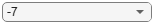"Train networks"; if trainingFlag == "Train networks" rawNet = trainNetwork(ds_Train_T,layers,options); else % Download the pre-trained networks modelsZipFile = matlab.internal.examples.downloadSupportFile('SPT','data/EEGEOGDenoisingNetworks.zip'); modelsFolder = fullfile(fileparts(datasetZipFile),'EEG_EOG_Denoising_Networks'); if ~exist(modelsFolder,'dir') unzip(modelsZipFile,fileparts(modelsZipFile)); end modelsFile = fullfile(modelsFolder,'trainedNetworks.mat'); load(modelsFile) end```### Analyze the Denoising Performance of the Trained Model

Use the test dataset to analyze the denoising performance of the `rawNet` network. Recall that the test dataset contains multiple test files for each SNR value in [-7, -6, -5, -4, -3, -2, -1, 0, 1, 2] dB. The performance metric is chosen as the mean-squared error (MSE) between the clean baseline EEG signal and the denoised EEG signal. The MSE of the clean EEG signal and the noisy EEG signal is also computed to show the worst-case MSE when no denoising is applied. At each SNR compute 340 MSE values for each of the 340 available test EEG segments and obtain the average MSE.

Create a `signalDatastore` to consume the test data and use a transformed datastore to setup data normalization. Since the data is now inside subfolders of the test folder, specify `IncludeSubfolders` as true. Further, use the folders2labels function to get the list of folder names for each file in the test dataset so that you can get data for each SNR.

```ds_Test = signalDatastore(fullfile(datasetFolder,"test"),SignalVariableNames=["noisyEEG","EEG"],IncludeSubfolders=true,ReadOutputOrientation="row"); ds_Test_T = transform(ds_Test,@normalizeData); % Get labels that contain the SNR value for each file in the datastore labels = folders2labels(ds_Test)```
```labels = 3400×1 categorical data_SNR_-1 data_SNR_-1 data_SNR_-1 data_SNR_-1 data_SNR_-1 data_SNR_-1 data_SNR_-1 data_SNR_-1 data_SNR_-1 data_SNR_-1 data_SNR_-1 data_SNR_-1 data_SNR_-1 data_SNR_-1 data_SNR_-1 data_SNR_-1 data_SNR_-1 data_SNR_-1 data_SNR_-1 data_SNR_-1 data_SNR_-1 data_SNR_-1 data_SNR_-1 data_SNR_-1 data_SNR_-1 data_SNR_-1 data_SNR_-1 data_SNR_-1 data_SNR_-1 data_SNR_-1 ⋮ ```

For each SNR value, denoise the test signals and compute the average MSE value. Use the `subset` function of the datastore to get a datastore pointing to the data for each SNR. To denoise a signal call the `predict` function passing the trained network and the noisy data as inputs.

```SNRs = (-7:2); MSE_Denoised_rawNet = zeros(numel(SNRs),1); % Measure denoising performance MSE_No_Denoise = zeros(numel(SNRs),1); % Measure worst-case MSE when no denoising is applied for idx = 1:numel(SNRs) lblIdx = find(labels == "data_SNR_"+num2str(SNRs(idx))); ds_Test_SNR = subset(ds_Test_T,lblIdx); % New datastore pointing to files with current SNR value % Denoise the data using the predict function of the trained model pred = predict(rawNet,ds_Test_SNR); % Use an array datastore to loop over the 340 denoised signals for the % current SNR value. Transform the datastore to add the normalization % step. ds_Pred = transform(arrayDatastore(pred,OutputType="same"),@normalizeData); mse = 0; mseWorstCase = 0; cnt = 0; while hasdata(ds_Pred) testData = read(ds_Test_SNR); denoisedData = read(ds_Pred); % MSE performance of denoiser - testData{2} contains clean EEG, % testData{1} contains noisy EEG. mse = mse + sum((testData{2} - denoisedData{1}).^2)/numel(denoisedData{1}); % Worst-case MSE performance when no denoising is applied. % Convert data to single precession as denoisedData is single % precision. mseWorstCase = mseWorstCase + sum((single(testData{2}) - single(testData{1})).^2)/numel(testData{1}); cnt = cnt+1; end % Average MSE of denoised signals MSE_Denoised_rawNet(idx) = mse/cnt; % Worst-case average MSE MSE_No_Denoise(idx) = mseWorstCase/cnt; end```

Plot the average MSE results.

```plot(SNRs,[MSE_No_Denoise,MSE_Denoised_rawNet],LineWidth=2) xlabel("SNR") ylabel("Average MSE") title("Denoising Performance") legend("Worst-case scenario (no-denoising)","Denoising with rawNet model")```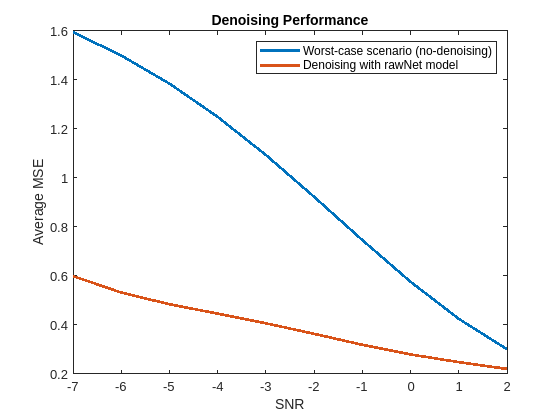### Improve Performance Using Short-Time Fourier Transform Feature Extraction

A common approach to improve performance of a deep learning model is to use extracted features in place of the original raw signal data. The features provide a representation of the input data that makes it easier for the network to learn the most important aspects of the signals.

Choose a short-time Fourier transformation (STFT) with a window length of 64 samples and overlap length of 63 samples. This transformation will effectively create 33 complex features with a length of 449 samples each. LSTM networks do not support complex inputs so the complex features can be separated into real and imaginary components by stacking the real part of the features on top of the imaginary part of the features to yield 66 real features each one of length 449 samples.

```winLength = 64; overlapLength = 63;```

The `transformSTFT` helper function listed at the end of this example normalizes the input signal and then computes its STFT. The function stacks the real and imaginary components to create a real output matrix. Further, if a GPU is available, the function moves the data to the GPU to accelerate the STFT computations and mitigate the increased complexity of computing the transforms. If you do not wish to use the GPU, set `useGPUFlag` to `false`.

`useGPUFlag =true;`

Compute and plot the STFT of a pair of clean and noisy EEG signals using the `transformSTFT` helper function.

```data = preview(ds_Train); P = transformSTFT(data,winLength,overlapLength,useGPUFlag); figure subplot(1,2,1) h = imagesc(P{2}); h.Parent.CLim = [-40 57]; title('STFT of clean EEG signal') ylabel("Stacked real and imaginary features") subplot(1,2,2) h = imagesc(P{1}); h.Parent.CLim = [-40 57]; ylabel("Stacked real and imaginary features") title('STFT of noisy EEG signal')```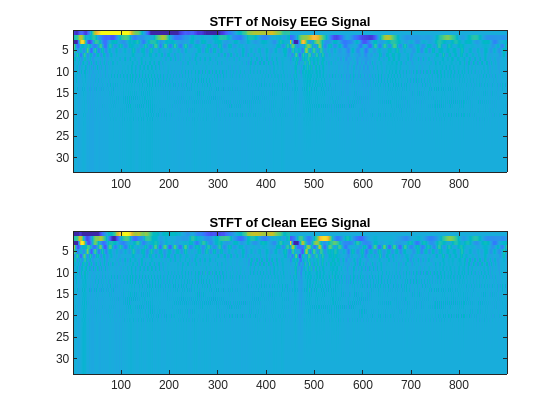The idea is to train a network so that it can produce denoised STFT signal representations based on STFT inputs corresponding to noisy signals. Note that the target outcome is a denoised signal, not its denoised STFT representation, so a final step must be added to compute the inverse STFT (ISTFT) to recover the denoised signal as depicted on the block diagram below.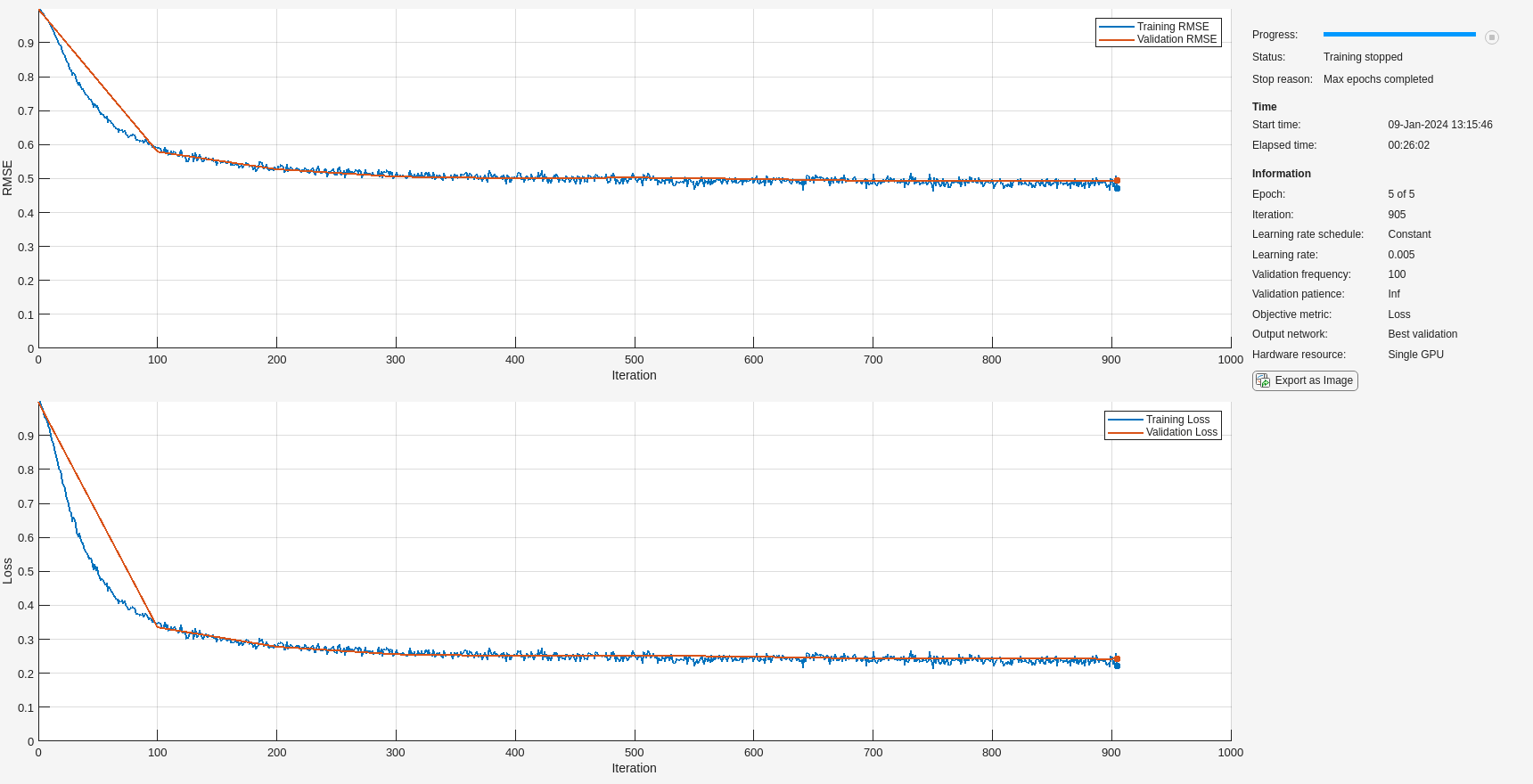The helper function, `transformISTFT`, listed at the end of this example takes the denoised STFT network output, converts the stacked real and imaginary features back to complex features and computes the inverse STFT. As a final step the function normalizes the resulting signal. If a GPU is available and `useGPUF`lag is true, the function performs all the computations in the GPU to reduce the processing time.

Create train, validation, and test datastores to apply STFT using the `transformSTFT` function.

```ds_Train_STFT = transform(ds_Train,@(d,wl,ol,fl)transformSTFT(d,winLength,overlapLength,useGPUFlag)); ds_Validate_STFT = transform(ds_Validate,@(d,wl,ol,fl)transformSTFT(d,winLength,overlapLength,useGPUFlag)); ds_Test_STFT = transform(ds_Test,@(d,wl,ol,fl)transformSTFT(d,winLength,overlapLength,useGPUFlag));```

Update the network architecture to account for 66 input and output features and specify the new validation data in the training options. Every other network parameter or option is unchanged.

```numFeatures = winLength + 2; layers = [ sequenceInputLayer(numFeatures) lstmLayer(numHiddenUnits) dropoutLayer(0.2) fullyConnectedLayer(numFeatures) regressionLayer]; options.ValidationData = ds_Validate_STFT;```

Train the network if `trainingFlag` is `"Train networks"`.

```if trainingFlag == "Train networks" stftNet = trainNetwork(ds_Train_STFT,layers,options); end```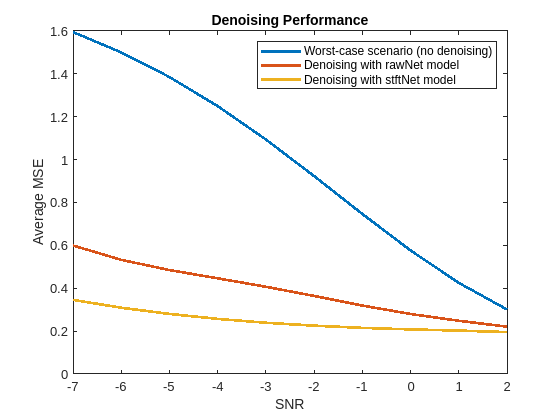Use the trained network to denoise EEG signals using the test data. Compute average MSE values by comparing denoised and clean baseline EEG signals.

```MSE_Denoised_stftNet = zeros(numel(SNRs),1); % Measure denoising performance for idx = 1:numel(SNRs) lblIdx = find(labels == "data_SNR_"+num2str(SNRs(idx))); % New datastores pointing to files with current SNR value ds_Test_SNR = subset(ds_Test_T,lblIdx); % Raw EEG signals to compute MSE ds_Test_STFT_SNR = subset(ds_Test_STFT,lblIdx); % STFT transforms % Denoise the data using the predict function of the trained model. pred = predict(stftNet,ds_Test_STFT_SNR); % Use an array datastore to loop over the 340 denoised signals for the % current SNR value. Transform the datastore to compute the inverse % STFT and recover the actual denoised signal. ds_Pred = transform(arrayDatastore(pred,OutputType="same"),@(P,wl,ol)transformISTFT(P,winLength,overlapLength)); mse = 0; cnt = 0; while hasdata(ds_Pred) testData = read(ds_Test_SNR); denoisedData = read(ds_Pred); % MSE performance of denoiser - testData{2} contains clean EEG mse = mse + sum((testData{2} - denoisedData).^2)/numel(denoisedData); cnt = cnt+1; end % Average MSE of denoised signals MSE_Denoised_stftNet(idx) = mse/cnt; end```

Plot the average MSE obtained with no denoising, denoising with a network trained with raw input signals, and denoising with a network trained with STFT transformed signals. You can see that the addition of the STFT step has improved the performance especially at the lower SNR values.

```figure plot(SNRs,[MSE_No_Denoise,MSE_Denoised_rawNet,MSE_Denoised_stftNet],LineWidth=2) xlabel("SNR") ylabel("Average MSE") title("Denoising Performance") legend("Worst-case scenario (no denoising)","Denoising with rawNet model","Denoising with stftNet model")```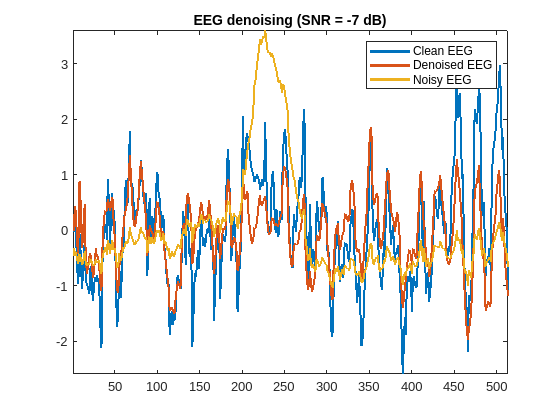Plot noisy and denoised signals for different SNRs. The `getRandomEEG` helper function listed at the end of this example gets a random EEG signal with a specified SNR from the test dataset.

```SNR =-7; % dB data = getRandomEEG(datasetFolder,SNR); noisyEEG = normalizeData(data{1}); cleanEEG = normalizeData(data{2}); stftInput = transformSTFT(noisyEEG,winLength,overlapLength,useGPUFlag); denoisedEEG = transformISTFT(predict(stftNet,stftInput),winLength,overlapLength); plot([cleanEEG.' denoisedEEG.' noisyEEG.'],LineWidth=2) title("EEG denoising (SNR = " + SNR + " dB)") legend("Clean EEG", "Denoised EEG","Noisy EEG") axis tight```### Conclusion

In this example you learned how to train a deep network to perform regression for signal denoising. You compared two models, one trained with raw clean and noisy EEG signals, the other trained with features extracted using a short-time Fourier transform. You learned that you can use complex features by stacking their real and imaginary components and treating them as independent real features. The use of STFT sequences provides greater performance improvement at worse SNRs and both approaches converge in performance as the SNR improves.

### References

 Haoming Zhang, Mingqi Zhao, Chen Wei, Dante Mantini, Zherui Li, Quanying Liu. "A benchmark dataset for deep learning solutions of EEG denoising." https://arxiv.org/abs/2009.11662

### Helper Functions

`normalizeData` - this function normalizes input signals by subtracting the mean and dividing by the standard deviation.

```function y = normalizeData(x) % This function is only intended to support examples in the Signal % Processing Toolbox. It may be changed or removed in a future release. if iscell(x) y = cell(1,numel(x)); y{1} = (x{1}-mean(x{1}))/std(x{1}); if numel(x) == 2 y{2} = (x{2}-mean(x{2}))/std(x{2}); end else y = (x - mean(x))/std(x); end end```

`transformSTFT` - this function normalizes the signals in input `data` and computes their short-time Fourier transform. It converts the complex STFT results into a real matrix by stacking the real and imaginary components one on top of the other.

```function P = transformSTFT(data,winLength,overlapLength,useGPUFlag) % This function is only intended to support examples in the Signal % Processing Toolbox. It may be changed or removed in a future release. if ~iscell(data) data = {data}; end P = cell(1,numel(data)); x = data{1}; if useGPUFlag x = gpuArray(x); end x = normalizeData(x); y = stft(x,Window=rectwin(winLength),OverlapLength=overlapLength,FrequencyRange="onesided"); P{1} = [real(y);imag(y)]; if numel(data) == 2 x = data{2}; if useGPUFlag x = gpuArray(x); end x = normalizeData(x); y = stft(x,Window=rectwin(winLength),OverlapLength=overlapLength,FrequencyRange="onesided"); P{2} = [real(y);imag(y)]; end end```

`transformISTFT` - this function takes a matrix with stacked real and imaginary STFT elements and combines them back to a complex STFT matrix. The function then computes the inverse STFT transform and normalizes the resulting reconstructed signals.

```function data = transformISTFT(P,winLength,overlapLength) % This function is only intended to support examples in the Signal % Processing Toolbox. It may be changed or removed in a future release. PP = P{1}; NumRows = size(PP,1); X = PP(1:NumRows/2,:)+1i*PP(1+NumRows/2:end,:); data = istft(X,Window=rectwin(winLength),OverlapLength=overlapLength,ConjugateSymmetric=true,FrequencyRange="onesided").'; data = normalizeData(data); end```

`createDataset` - this function combines clean EEG signal segments with EOG segments to create training, validation and testing datasets to train an EEG denoiser neural network.

```function createDataset(dataDir) % This function is only intended to support examples in the Signal % Processing Toolbox. It may be changed or removed in a future release. % Create training, validation, and testing datasets consisting of clean EEG % signals and noisy EEG signals contaminated by EOG segments. load(fullfile(dataDir,"EEG_all_epochs.mat"),"EEG_all_epochs"); load(fullfile(dataDir,"EOG_all_epochs.mat"),"EOG_all_epochs"); EEG_all_epochs = EEG_all_epochs(1:3400,:).'; EOG_all_epochs = EOG_all_epochs.'; Fs = 256; trainingPercentage = 80; validationPercentage = 10; N = size(EEG_all_epochs,2); % Create a training dataset consisting of mat files containing two signals % - a clean EEG signal, and an EEG signal contaminated by EOG artifacts. % Combine each of 2720 pairs of EEG and EOG segments ten times with random % SNRs in the range -7dB to 2dB to obtain 27200 training segments. EEG_training = EEG_all_epochs(:,1:N*trainingPercentage/100); EOG_training = EOG_all_epochs(:,1:N*trainingPercentage/100); M = size(EEG_training,2); cnt = 0; if ~exist(fullfile(dataDir,"train"),'dir') mkdir(fullfile(dataDir,"train")) end for idx = 1:M for kk = 1:10 cnt = cnt + 1; EEG = EEG_training(:,idx).'; EOG = EOG_training(:,idx).'; [noisyEEG,SNR] = createNoisySegment(EEG,EOG,[-7,2]); save(fullfile(dataDir,"train","data_" + num2str(cnt) + ".mat"),"EEG","EOG","noisyEEG","Fs","SNR"); end end % Create a validation dataset by combining 340 pairs of EEG and EOG % segments ten times with random SNRs in (-7:2) dB EEG_validation = EEG_all_epochs(:,1+N*trainingPercentage/100:N*trainingPercentage/100+N*validationPercentage/100); EOG_validation = EOG_all_epochs(:,1+N*trainingPercentage/100:N*trainingPercentage/100+N*validationPercentage/100); M = size(EEG_validation,2); cnt = 0; if ~exist(fullfile(dataDir,"validate"),'dir') mkdir(fullfile(dataDir,"validate")) end for idx = 1:M for kk = 1:10 cnt = cnt + 1; EEG = EEG_validation(:,idx).'; EOG = EOG_validation(:,idx).'; [noisyEEG,SNR] = createNoisySegment(EEG,EOG,[-7,2]); save(fullfile(dataDir,"validate","data_" + num2str(cnt) + ".mat"),"EEG","EOG","noisyEEG","Fs","SNR"); end end % Create a test dataset by combining 340 pairs of EEG and EOG segments ten % times with 10 SNR values [-7 -6 -5 -4 -3 -2 -1 0 1 2] dB. Store the % training sets in folders with names that identify the SNR value so that % it is easy to analyze performance by accessing files with a specific SNR. EEG_test = EEG_all_epochs(:,1+N*trainingPercentage/100+N*validationPercentage/100:end); EOG_test = EOG_all_epochs(:,1+N*trainingPercentage/100+N*validationPercentage/100:end); M = size(EEG_test,2); SNRVect = (-7:2); for kk = 1:numel(SNRVect) cnt = 0; if ~exist(fullfile(dataDir,"test","data_SNR_" + num2str(SNRVect(kk))),'dir') mkdir(fullfile(dataDir,"test","data_SNR_" + num2str(SNRVect(kk)))); end for idx = 1:M cnt = cnt + 1; EEG = EEG_test(:,idx).'; EOG = EOG_test(:,idx).'; [noisyEEG,SNR] = createNoisySegment(EEG,EOG,SNRVect(kk)); save(fullfile(dataDir,"test","data_SNR_" + num2str(SNR)+"/" + "data_"+num2str(cnt) + ".mat"),"EEG","EOG","noisyEEG","Fs","SNR"); end end end function [y,SNROut] = createNoisySegment(eeg,artifact,SNR) % Combine EEG and artifact signals with a specified SNR in dB. If SNR is a % two-element vector, its value is chosen randomly from a uniform % distribution over the interval [SNR(1) SNR(2)] if numel(SNR) == 2 SNR = SNR(1) + (SNR(2)-SNR(1)).*rand(1,1); end k = 10^(SNR/10); lambda = (1/k)*rms(eeg)/rms(artifact); y = eeg + lambda * artifact; SNROut = SNR; end```

`getRandomEEG -`` this function reads the data from a `random EEG test file with a specified SNR.

```function data = getRandomEEG(datasetFolder,SNR) sds = signalDatastore(fullfile(datasetFolder,"test","data_SNR_"+num2str(SNR)),SignalVariableNames=["noisyEEG","EEG"],IncludeSubfolders=true); n = numel(sds.Files); idx = randi(n,1); data = read(subset(sds,idx)); end```